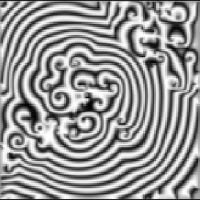#Boost C++ Libraries

...one of the most highly regarded and expertly designed C++ library projects in the world.

This is the documentation for an old version of Boost. Click here to view this page for the latest version.Home Libraries People FAQ More

### Using matrices as state types

odeint works well with a variety of different state types. It is not restricted to pure vector-wise types, like `vector< double >`, `array< double , N >`, `fusion::vector< double , double >`, etc. but also works with types having a different topology then simple vectors. Here, we show how odeint can be used with matrices as states type, in the next section we will show how can be used to solve ODEs defined on complex networks.

By default, odeint can be used with `ublas::matrix< T >` as state type for matrices. A simple example is a two-dimensional lattice of coupled phase oscillators. Other matrix types like `mtl::dense_matrix` or blitz arrays and matrices can used as well but need some kind of activation in order to work with odeint. This activation is described in following sections,

The definition of the system is

```typedef boost::numeric::ublas::matrix< double > state_type;

struct two_dimensional_phase_lattice
{
two_dimensional_phase_lattice( double gamma = 0.5 )
: m_gamma( gamma ) { }

void operator()( const state_type &x , state_type &dxdt , double /* t */ ) const
{
size_t size1 = x.size1() , size2 = x.size2();

for( size_t i=1 ; i<size1-1 ; ++i )
{
for( size_t j=1 ; j<size2-1 ; ++j )
{
dxdt( i , j ) =
coupling_func( x( i + 1 , j ) - x( i , j ) ) +
coupling_func( x( i - 1 , j ) - x( i , j ) ) +
coupling_func( x( i , j + 1 ) - x( i , j ) ) +
coupling_func( x( i , j - 1 ) - x( i , j ) );
}
}

for( size_t i=0 ; i<x.size1() ; ++i ) dxdt( i , 0 ) = dxdt( i , x.size2() -1 ) = 0.0;
for( size_t j=0 ; j<x.size2() ; ++j ) dxdt( 0 , j ) = dxdt( x.size1() -1 , j ) = 0.0;
}

double coupling_func( double x ) const
{
return sin( x ) - m_gamma * ( 1.0 - cos( x ) );
}

double m_gamma;
};
```

In principle this is all. Please note, that the above code is far from being optimal. Better performance can be achieved if every interaction is only calculated once and iterators for columns and rows are used. Below are some visualizations of the evolution of this lattice equation.The full cpp for this example can be found here two_dimensional_phase_lattice.cpp.

 Copyright © 2009-2015 Karsten Ahnert and Mario Mulansky Distributed under the Boost Software License, Version 1.0. (See accompanying file LICENSE_1_0.txt or copy at http://www.boost.org/LICENSE_1_0.txt)